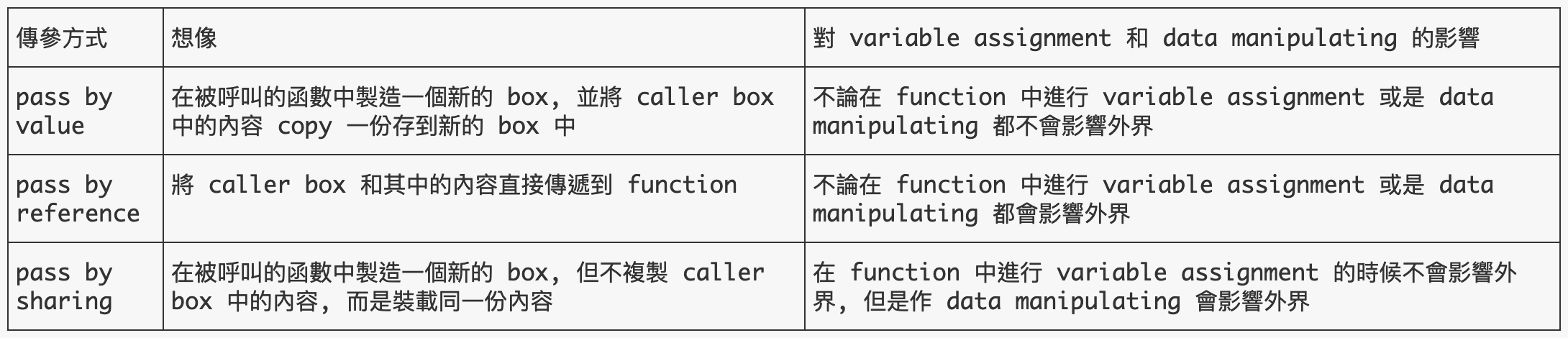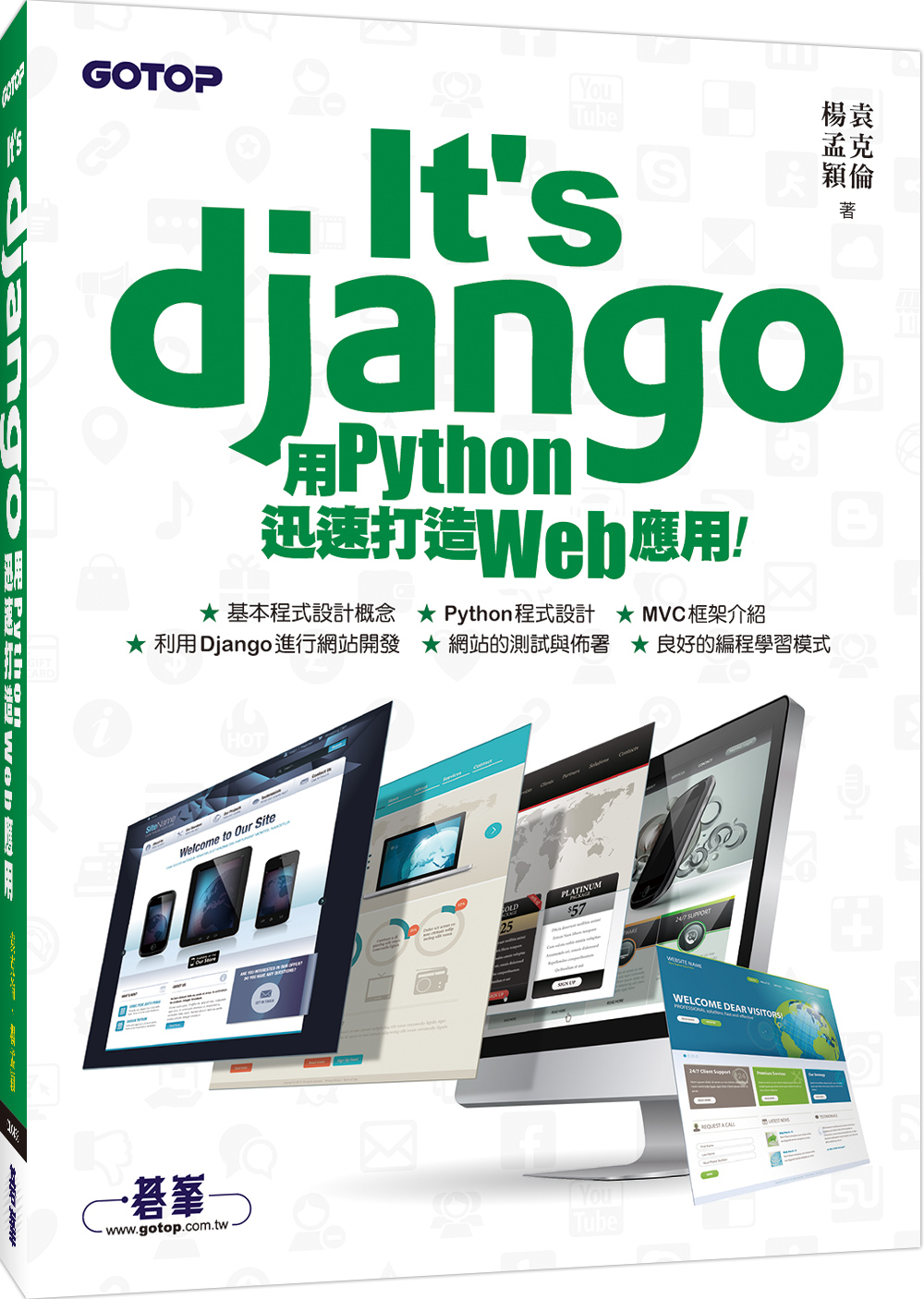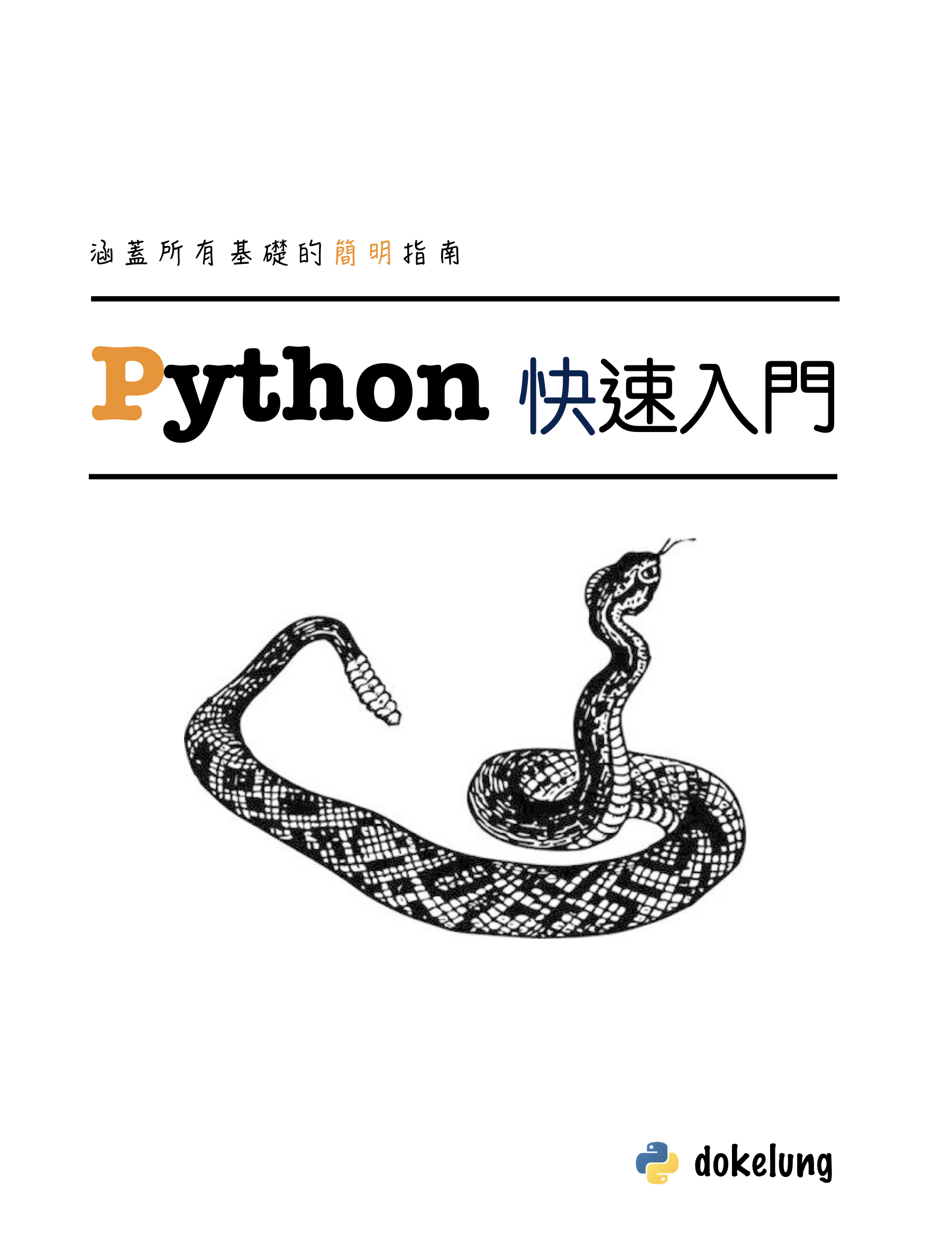• home
• category
• python
• Python 到底是 pass by value 還是 pass by reference? 一次搞懂程式語言的函式傳參!

# Python 到底是 pass by value 還是 pass by reference? 一次搞懂程式語言的函式傳參!## 原本的問題

```class Count:
def __init__(self, count=0):
self.count = count

def increment(c, times):
c.count += 1
print('c:', c.count)
times += 1
print('t:', times)

def main():
c = Count()
times = 0
for i in range(100):
increment(c, times)

main()
```

```c: 97
t: 1
c: 98
t: 1
c: 99
t: 1
c: 100
t: 1
```

1. 認為 Python 是 pass by value 或是 pass by reference (你也這樣想嗎？那我建議你看下去！)
2. 認為 Python 會根據所傳入物件是可變還是不可變的去選擇使用 pass by value 和 pass by reference (這下扯更遠了)
3. 想要使用 built-in function `id` 來做佐證，但沒有搞清楚 `id` 問的是誰的 id

## (1) 從 variable 跟 value 談起

variable 是一個抽象的概念，value 是一個實際存在的資料

variable 不是其對應的 value，vairable 儲存(參考)其對應的 value

```a = 5
```

variable 跟 value 是兩回事, 但他們有 指涉關係

## (2) 關於 buildin function `id`

`id` 常常會有直覺上的誤解, 因為我們常常會使用 variable 作為 `id` 的 argument:

```>>> a = 5
>>> id(a)
140035495798656
>>> a = 6
>>> id(a)
140035495798688
>>> id(5)
140035495798656
>>> id(6)
140035495798688
```

`id` 問的是 value 的 id 不是 variable 的 id

## (3) variable assignment 與 data manipulating

(3) 這個標題中提到兩種不同的動作(這是我自己亂叫的, 並非專有名詞, 慎之):

variable assignment 指的是變數的指派跟重新繫結:

```>>> a = []
>>> id(a)
140035460871496
>>> a = [1, 2, 3]
>>> id(a)
140035460871560
```

data manipulating 指的是 data 本身進行了一些操作, 例如:

```>>> a = []
>>> id(a)
140035460871496
>>> a.extend([1, 2, 3])
>>> id(a)
140035460871496
```

P.S. 關於這兩種概念的討論和注意事項請見 SegmentFault 網站該問題的 評論 處, 我與網友 chaneyzorn 的討論。

## (4) 函數調用時的傳遞方式

1. pass by value
2. pass by reference
3. pass by sharing (call by object reference)

### pass by value

```void foo(int a) {
a = 6;
return;
}

int main() {
int a = 1;
foo(a);
return 0;
}
```

### pass by reference

```void foo(int& a) {
a = 6;
return;
}

int main() {
int a = 1;
foo(a);
return 0;
}
```

```void modify(int p, int* q, int* r) {
p = 27;  // passed by value: only the local parameter is modified
*q = 27; // passed by value or reference, check call site to determine which
*r = 27; // passed by value or reference, check call site to determine which
}

int main() {
int a = 1;
int b = 1;
int x = 1;
int* c = &x;

// a is passed by value
// b is passed by reference by creating a pointer
// c is a pointer passed by value
// b and x are changed
modify(a, &b, c);

return 0;
}
```

### pass by sharing

```def foo(lst):
lst.append(1)
lst = 

m = []
foo(m)
print(m)
```

## (5) 回頭看本題

```class Count:
def __init__(self, count=0):
self.count = count

def increment(c, times):
c.count += 1
# print('c:', c.count)
print('c:', id(c))
times += 1
# print('t:', times)
print('t:', id(times))

if __name__ == '__main__':
c = Count()
times = 0
print('times:', id(times))
for i in range(100):
increment(c, times)
```

```def increment(c, times):
print('t begin:', id(times))
c.count += 1
...
```

```times: 140303106782944
t begin: 140303106782944
c: 140303062368832
t: 140303106782976
t begin: 140303106782944
c: 140303062368832
t: 140303106782976
t begin: 140303106782944
c: 140303062368832
t: 140303106782976
t begin: 140303106782944
```

## (6) 結論## (7) 參考資料

### dokelungHi, my name is Ko-Lung Yuan!

book

### It's djangoA book about python web framework Django

book

### Python 快速入門